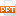# Toán học - Chapter 11: Trees

Theorem: An undirected graph is a tree if and only if there is a unique simple path between any two of its vertices. Proof: Assume that T is a tree. Then T is connected with no simple circuits. Hence, if x and y are distinct vertices of T, there is a simple path between them (by Theorem 1 of Section 10.4). This path must be unique - for if there were a second path, there would be a simple circuit in T (by Exercise 59 of Section 10.4). Hence, there is a unique simple path between any two vertices of a tree. Now assume that there is a unique simple path between any two vertices of a graph T. Then T is connected because there is a path between any two of its vertices. Furthermore, T can have no simple circuits since if there were a simple circuit, there would be two paths between some two vertices. Hence, a graph with a unique simple path between any two vertices is a tree.43 trang | Chia sẻ: huyhoang44 | Ngày: 19/03/2020 | Lượt xem: 19 | Lượt tải: 0
Bạn đang xem trước 20 trang tài liệu Toán học - Chapter 11: Trees, để xem tài liệu hoàn chỉnh bạn click vào nút DOWNLOAD ở trên
•chapter11_007.pptx